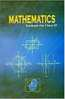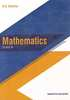# Textbook Solutions for CBSE (Arts) Class 11 - CBSE - Mathematics

Subjects
Subjects
Popular subjects
Mathematics
< prev 1 to 2 of 2 next >Author: NCERT
Publisher: NCERT

16 Chapters:
1 Sets
2 Relations and Functions
3 Trigonometric Functions
4 Principle of Mathematical Induction
5 Complex Numbers and Quadratic Equations
6 Linear Inequalities
7 Permutations and Combinations
8 Binomial Theorem
9 Sequences and Series
10 Straight Lines
11 Conic Sections
12 Introduction to Three Dimensional Geometry
13 Limits and Derivatives
14 Mathematical Reasoning
15 Statistics
16 ProbabilityAuthor: r.d.sharma
Publisher: Dhanpat Rai Publications

33 Chapters:
1 Sets
2 Relations
3 Functions
4 Measurement of Angles
5 Trigonometric Functions
6 Graphs of Trigonometric Functions
7 Values of Trigonometric function at sum or difference of angles
8 Transformation formulae
9 Values of Trigonometric function at multiples and submultiples of an angle
10 Sine and cosine formulae and their applications
11 Trigonometric equations
12 Mathematical Induction
13 Complex Numbers
15 Linear Inequations
16 Permutations
17 Combinations
18 Binomial Theorem
19 Arithmetic Progression
20 Geometric Progression
21 Some special series
22 Brief review of cartesian system of rectangular co-ordinates
23 The straight lines
24 The circle
25 Parabola
26 Ellipse
27 Hyperbola
28 Introduction to three dimensional coordinate geometry
29 Limits
30 Derivatives
31 Mathematical reasoning
32 Statistics
33 Probability

< prev 1 to 2 of 2 next >

## Textbook solutions and Reference book solutions for Class 11

• On this page you will find NCERT solution, RD Sharma solution for Class 11.
• Each chapter comes with subsections that provide in-depth knowledge about the basic concepts.
• Solved equations along with examples help the students to understand the different steps towards solving their exercises.
• Compilation of different exercises makes it a great choice for students to practice to brush up their concepts and skills.
• These books are a must-have for the students who want to closely evaluate the different aspects of their respective subjects.
• Different graphs along with schematic representations help the students to memorise the many steps.
• These solutions for Class 11 are prepared by experts, include the problems from latest edition and are as per CBSE pattern.

## Reference book solutions and textbook solutions on Shaalaa.com

Shaalaa.com is a great source for Reference book solutions and textbook solutions of all Subjects for Practice. Our best teachers have reviewed all the Class 11 solutions of exercise questions that will help you solve all the Class 11 questions of all subject without any problem. Reference book Solutions are highly beneficial especially when you are thinking to prepare for school as well as competitive level examinations. We at Shaalaa.com work hard to create better ways in order to help students and provide you with the detailed solutions for all questions from reference boook and textbook as per the latest marking scheme pattern is to back you up in effective learning in all the examinations conducted by CBSE.